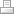Glen Hills Primary School

Featherby Drive

Glen Parva﻿

Leicester

LE2 9NY

﻿

Tel: 0116 2782535

Fax: 0116 2788208

Registered Company Number 07941899

Glen Hills Primary School is a Trust member and Lead Hub school for the Symphony Learning Trust

Maths

Maths Coordinator:  Mrs Janette de Voil

Maths Statement of Intent
Maths Intent Statement.pdf

Maths Policy
Maths Policy 2019 (1).pdf

Maths - Addition and Subtraction - Progression Map

Maths - Algebra - Progression Map
Maths Algebra Progression Map.pdf

Maths - Fractions - Progression Map
Maths Fractions Progression Map.pdf

Maths - Geometry: Position and Direction - Progression Map
Maths Geometry Position and Direction Pr[...]

Maths - Geometry: Properties of Shape - Progression Map
Maths Geometry Properties of Shape Progr[...]

Maths - Measurement - Progression Map
Maths Measurement Progression Map.pdf

Maths - Multiplication and Division - Progression Map
Maths Multiplication and Division Progre[...]

Maths - Number and Place Value - Progression Map
Maths Number and Place Value Progression[...]

Maths - Ratio and Proportion - Progression Map
Maths Ratio and Proportion Progression M[...]

Maths - Statistics - Progression Map
Maths Statistics Progression Map.pdfPrint | Sitemap